Surface Area
Volume
Cross Sections
Word Problems and Applications
Formulas
100

Find the surface area of the rectangular prism.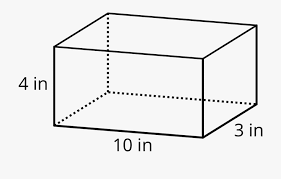164 square inches.

100

Find the volume of the rectangular prism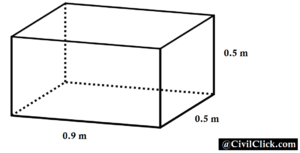0.225 cubic meters

100

The horizontal cross section of a cylinder: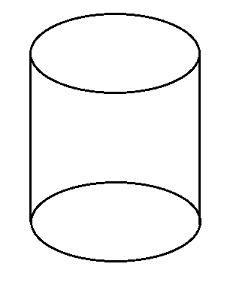Circle

100

A room is 144 square feet. If tile costs $0.83 per square foot, how much would it cost to put tile in?$119.52

100

1/2 h (b1 + b2)

What is area of a trapezoid?

200

Find the surface area of the rectangular prism.2.3 square meters

200

Find the volume of the rectangular pyramid.224 in3

200

The vertical cross section of a cylinder:Rectangle

200

A gallon of paint can cover 45 square feet. If a wall is 325 square feet, how many gallons of paint should you buy?

8 gallons

200

1/2 * b * h

What is area of a triangle?

300

Find the surface area of the triangular prism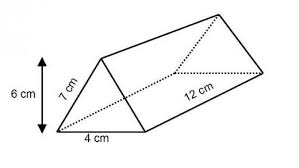240 square centimeters.

300

Find the volume of the triangular prism144 cubic centimeters

300

The vertical cross section of a cone, through its apex: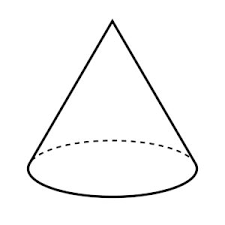Triangle

300

You are filling the rectangular pyramid with sand. How many cubic inches of sand will you need?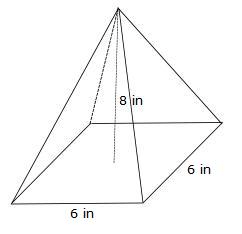96 cubic inches

300

b*h

What is area of a parallelogram?

400

Find the surface area of the square pyramid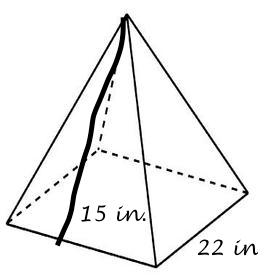1,144 in2

400

Find the volume of the cylinder. Use 3.14 for pi.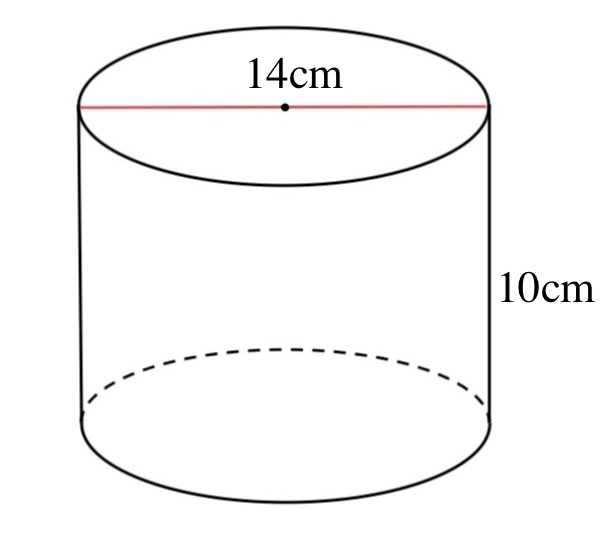1,538.6 cm3

400

What is the largest cross-section that could result from slicing a rectangular prism?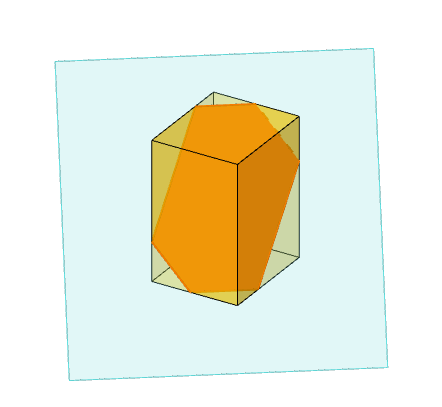Hexagon

400

If it costs $0.25 per square inch to paint this prism, approximately how much will it cost?$30

400

1/3 B*h

What is volume of a pyramid?

500

Find the surface area of the trapezoidal prism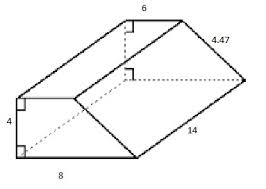506.58 square units

500

Find the volume of the trapezoidal prism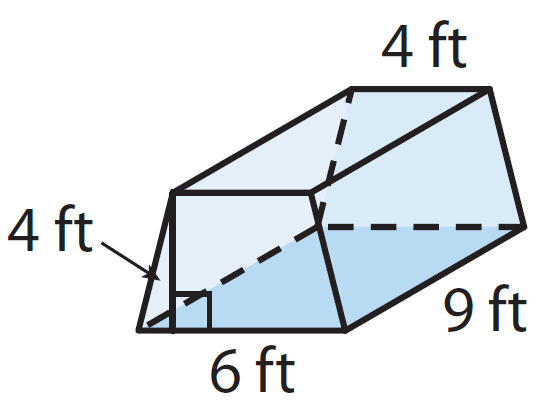180 cubic feet

500

If a cone was sliced at an angle, what would be the resulting cross-section?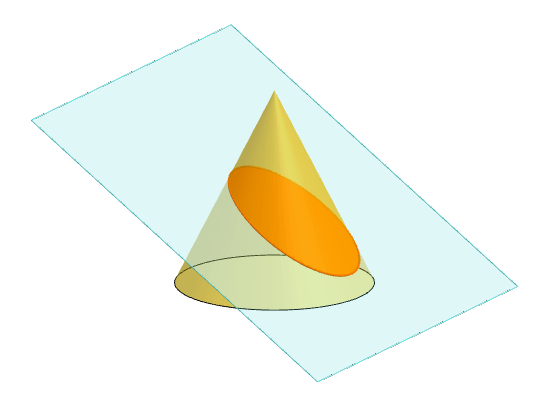Oval (bonus points if you said ellipse)

500

The following prism is being filled with sand. Sand costs $2.25 per square foot. How much will in cost?$405

500

B*h

What is volume of a prism?

Click to zoom